# Circle Graph Worksheet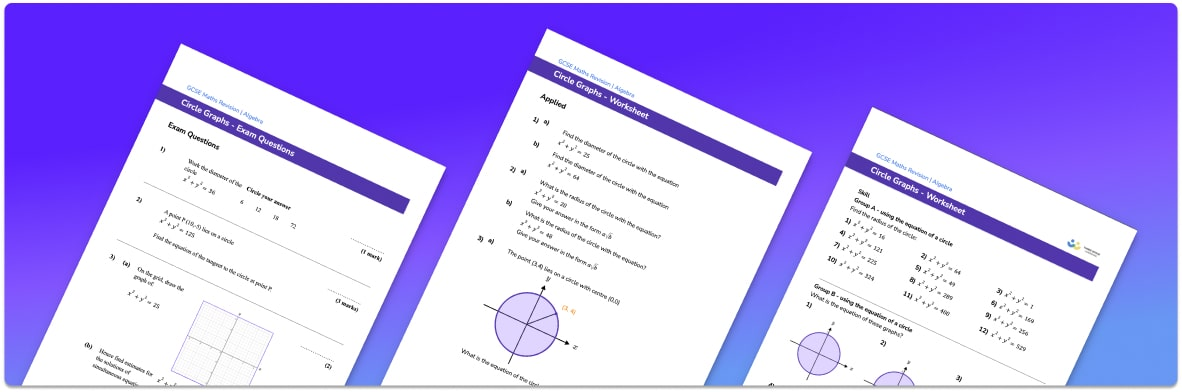• Section 1 of the circle graph worksheet contains 20+ skills-based  circle graph questions, in 3 groups to support differentiation
• Section 2 contains 3 applied circle graph questions with a mix of worded problems and deeper problem solving questions
• Section 3 contains 3 foundation and higher level GCSE exam style circle graph questions
• Answer keys and a mark scheme for all circle graph questions are provided
• Questions follow variation theory with plenty of opportunities for students to work independently at their own level
• All questions created by fully qualified expert secondary maths teachers
• Suitable for GCSE maths revision for AQA, OCR and Edexcel exam boards

• This field is for validation purposes and should be left unchanged.

You can unsubscribe at any time (each email we send will contain an easy way to unsubscribe). To find out more about how we use your data, see our privacy policy.

### Circle graph at a glance

Circle graphs with a centre (0, 0) have the general equation x2+y2=r2, where r is the radius of the circle.

To draw a circle graph we look at the radius and draw a circle with the given radius around the centre (0, 0). If we are given a point on a circle graph, we can find the equation of the circle by finding the length of the radius using Pythagoras’ theorem. We can then use this to write the equation of the circle.

Given a point on a circle we can also find the gradient of the radius. We can use this to find the gradient of the tangent (which is perpendicular to the radius) and therefore the equation of the tangent to the circle.

We can use circle graphs to solve pairs of simultaneous equations where one is the equation of a circle. If we draw the graphs of both equations, the points of intersection between the line and the circle graph show the solutions to the simultaneous equations.

Looking forward, students can then progress to additional algebra worksheets, for example a simplifying expressions worksheet or simultaneous equations worksheet.For more teaching and learning support on Algebra our GCSE maths lessons provide step by step support for all GCSE maths concepts.

## Related worksheets

Simplifying Algebraic Fractions Worksheet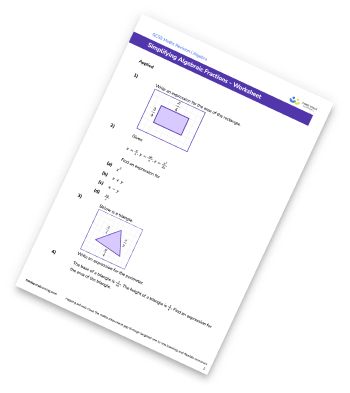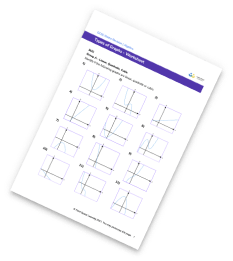Parallel And Perpendicular Lines Worksheet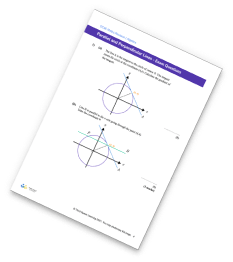Y=Mx+C Worksheet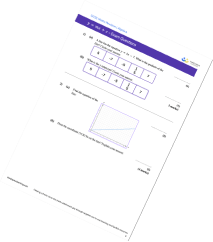## Do you have KS4 students who need more focused attention to succeed at GCSE?There will be students in your class who require individual attention to help them succeed in their maths GCSEs. In a class of 30, it’s not always easy to provide.

Help your students feel confident with exam-style questions and the strategies they’ll need to answer them correctly with our dedicated GCSE maths revision programme.

Lessons are selected to provide support where each student needs it most, and specially-trained GCSE maths tutors adapt the pitch and pace of each lesson. This ensures a personalised revision programme that raises grades and boosts confidence.

Find out more